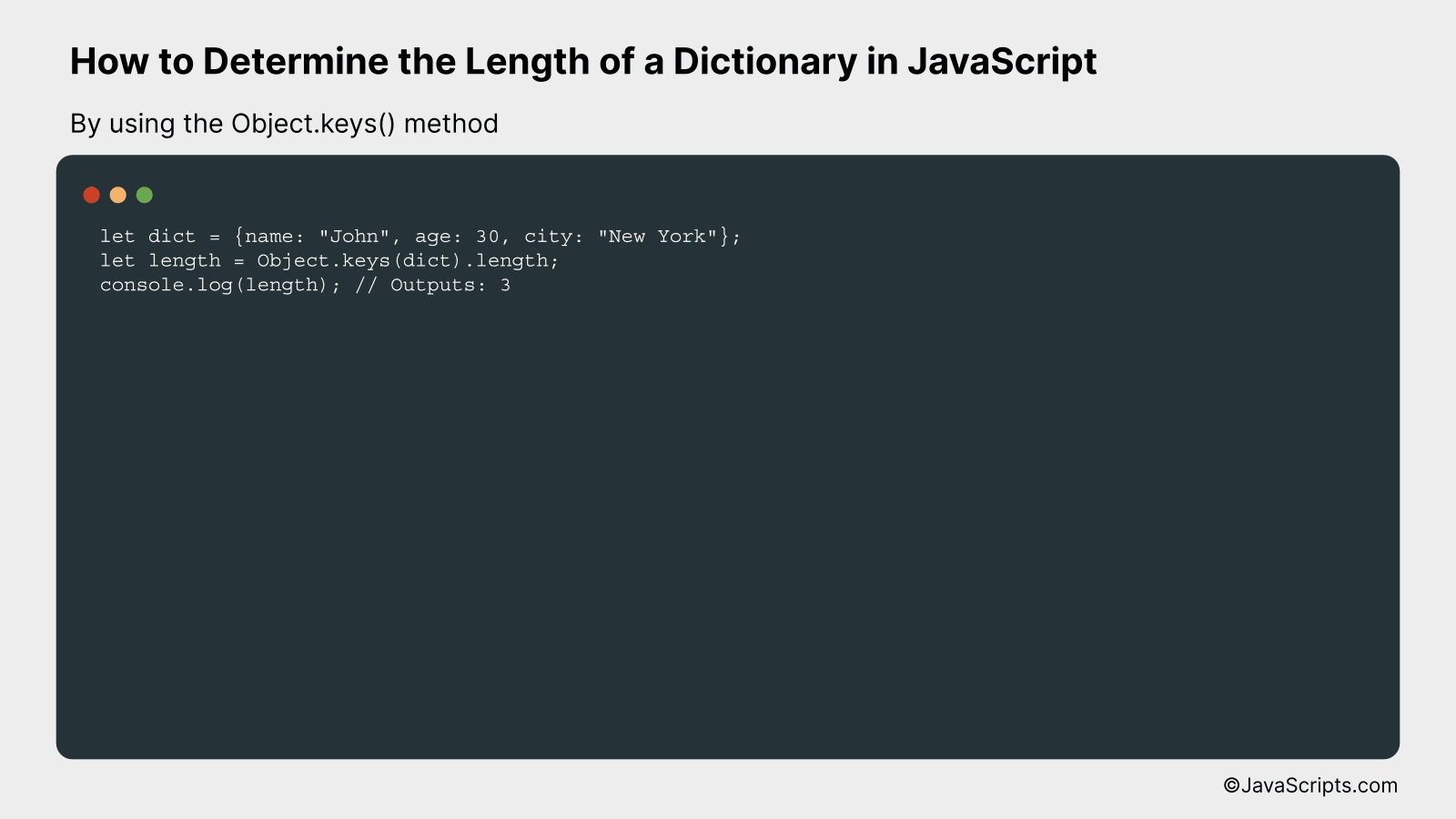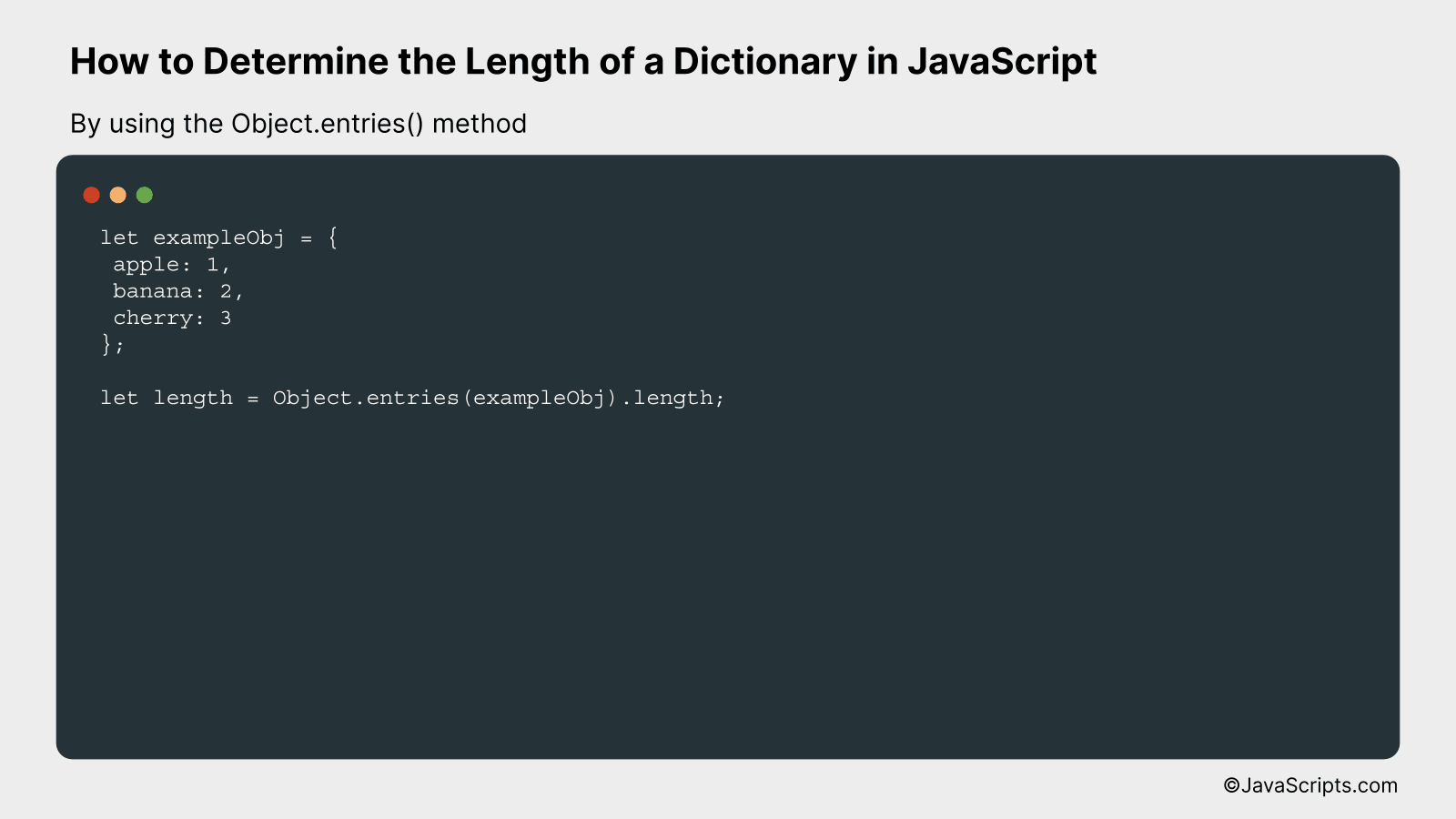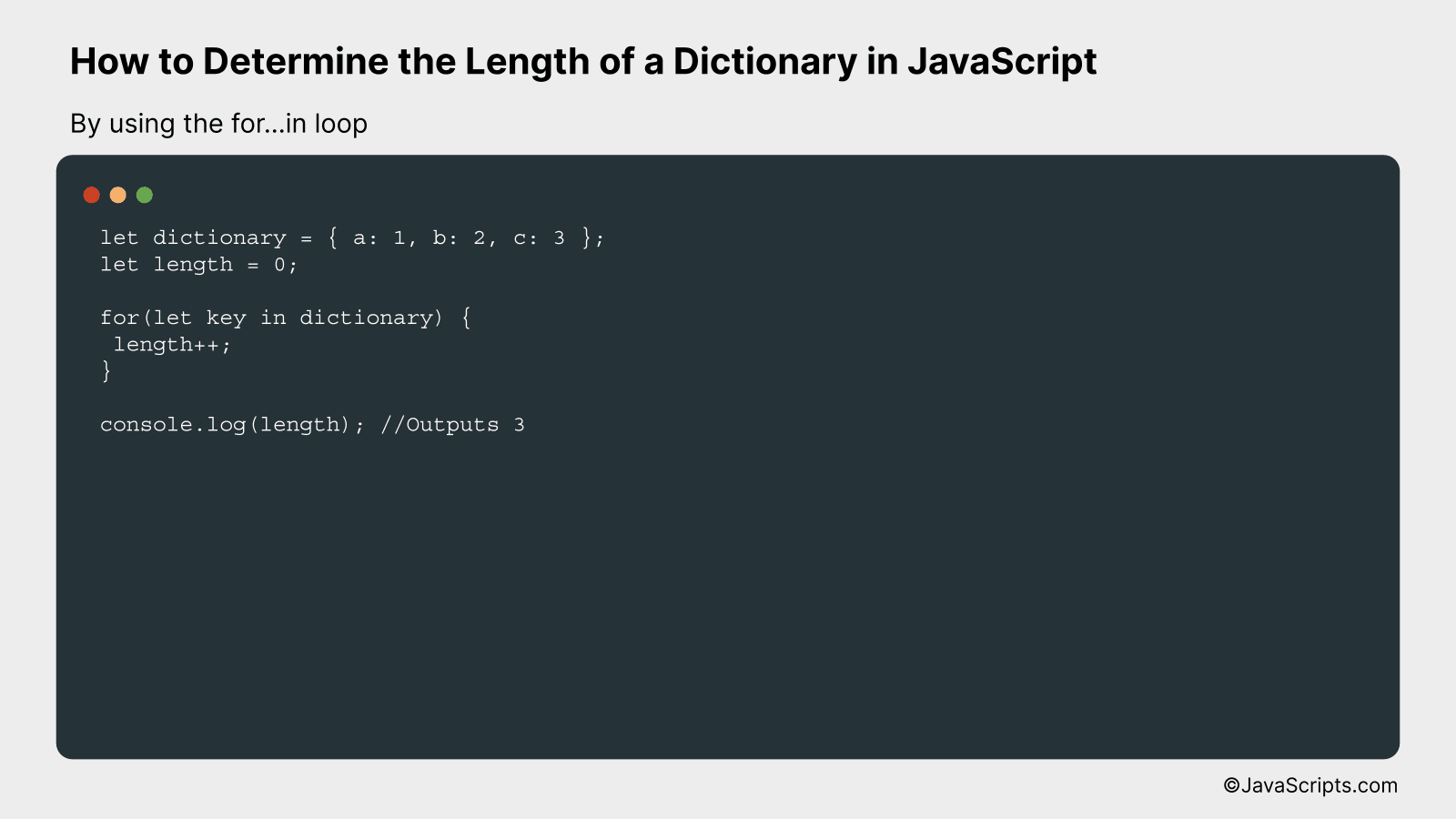# How to Determine the Length of a Dictionary in JavaScript

Navigating JavaScript can be quite an adventure, especially when it comes to handling and understanding its versatile data types. One of the most powerful tools at our disposal is the dictionary, or object, a collection of key-value pairs that can hold a wide range of data types.

Now, have you ever found yourself stuck, wondering how many key-value pairs you have in your dictionary? We’ve all been there. Today, we’ll break down the process of determining the length of a dictionary in JavaScript. It’s simpler than you might think and by the end, you’ll have a new tool in your coding toolbox.

In this post, we will be looking at the following 3 ways to determine the length of a dictionary in JavaScript:

• By using the Object.keys() method
• By using the Object.entries() method
• By using the for…in loop

Let’s explore each…

## #1 – By using the Object.keys() methodThe Object.keys() method in JavaScript returns an array of a given object’s own enumerable property names. You can determine the length of a dictionary (object) by calling this method on the object and then checking the length of the returned array. This is best understood through an example.

``````
let dict = {name: "John", age: 30, city: "New York"};
let length = Object.keys(dict).length;
console.log(length); // Outputs: 3
``````

### How it works

The Object.keys() method retrieves all the enumerable properties of a JavaScript object in the form of an array. This array’s length property is then used to determine the number of keys in the dictionary.

• Step 1: Declare and initialize an object (dictionary). For instance, here we’re using dict = {name: “John”, age: 30, city: “New York”}.
• Step 2: Use the Object.keys(dict) method, which will return an array of the object’s keys. For our object, it’ll return [‘name’, ‘age’, ‘city’].
• Step 3: Use the length property on the array obtained from Step 2. This gives us the count of keys in the object, which represents the length of the dictionary. For our object, it’s 3.
• Step 4: Print the value of length to the console using console.log(length). This will output 3, the length of our dictionary.

## #2 – By using the Object.entries() methodThe JavaScript method Object.entries() can be used to determine the length of a dictionary (object) by returning an array of the object’s enumerable property [key, value] pairs which can then be counted with the .length property. Let’s understand this in detail with an example:

``````
let exampleObj = {
apple: 1,
banana: 2,
cherry: 3
};

let length = Object.entries(exampleObj).length;
``````

### How it works

The Object.entries() method returns an array whose elements are arrays corresponding to the enumerable property [key, value] pairs found directly upon the object. The .length property of this array gives us the count of these pairs, which is essentially the number of properties in the original object.

• First, we define an object exampleObj with 3 properties: apple, banana, and cherry.
• Next, Object.entries(exampleObj) returns an array of [key, value] pairs for the object, which looks like this: [[‘apple’, 1], [‘banana’, 2], [‘cherry’, 3]].
• Then, the .length property of this array is accessed, which gives us the count of these pairs, and hence, the number of properties in the exampleObj. In this case, it is 3.
• This count is stored in the variable length.

## #3 – By using the for…in loopIn JavaScript, you can determine the length of a dictionary (an object in JavaScript) using a for…in loop, by incrementing a counter for each property in the object. Let’s understand this with a code sample.

``````
let dictionary = { a: 1, b: 2, c: 3 };
let length = 0;

for(let key in dictionary) {
length++;
}

console.log(length); //Outputs 3
``````

### How it works

The for…in loop in JavaScript iterates over all enumerable properties of an object. In the given code, we are using this loop to find the count of properties (or ‘keys’), which is essentially the length of the dictionary.

• First, we define the dictionary (object) with three properties: a, b, and c.
• Next, we initialize a variable ‘length’ to 0. This will be used to count the number of properties in the dictionary.
• We then create a for…in loop with ‘key’ as the variable that will take on the name of each property in the dictionary. For each property, we increment the ‘length’ variable by 1.
• After the loop has iterated over all properties, ‘length’ will hold the total count of properties, which is the length of the dictionary.
• Finally, we output the value of ‘length’ using console.log, which in this case will output 3.

Related:

In conclusion, finding the length of a dictionary in JavaScript is quite easy. It only needs a basic knowledge of the ‘Object.keys()’ method, which quickly returns an array of keys present in the dictionary.

Remember, the dictionary in JavaScript is an object with key-value pairs. To determine its length, first convert the dictionary into an array using ‘Object.keys()’, then find the array’s length. That’s all there is to it.